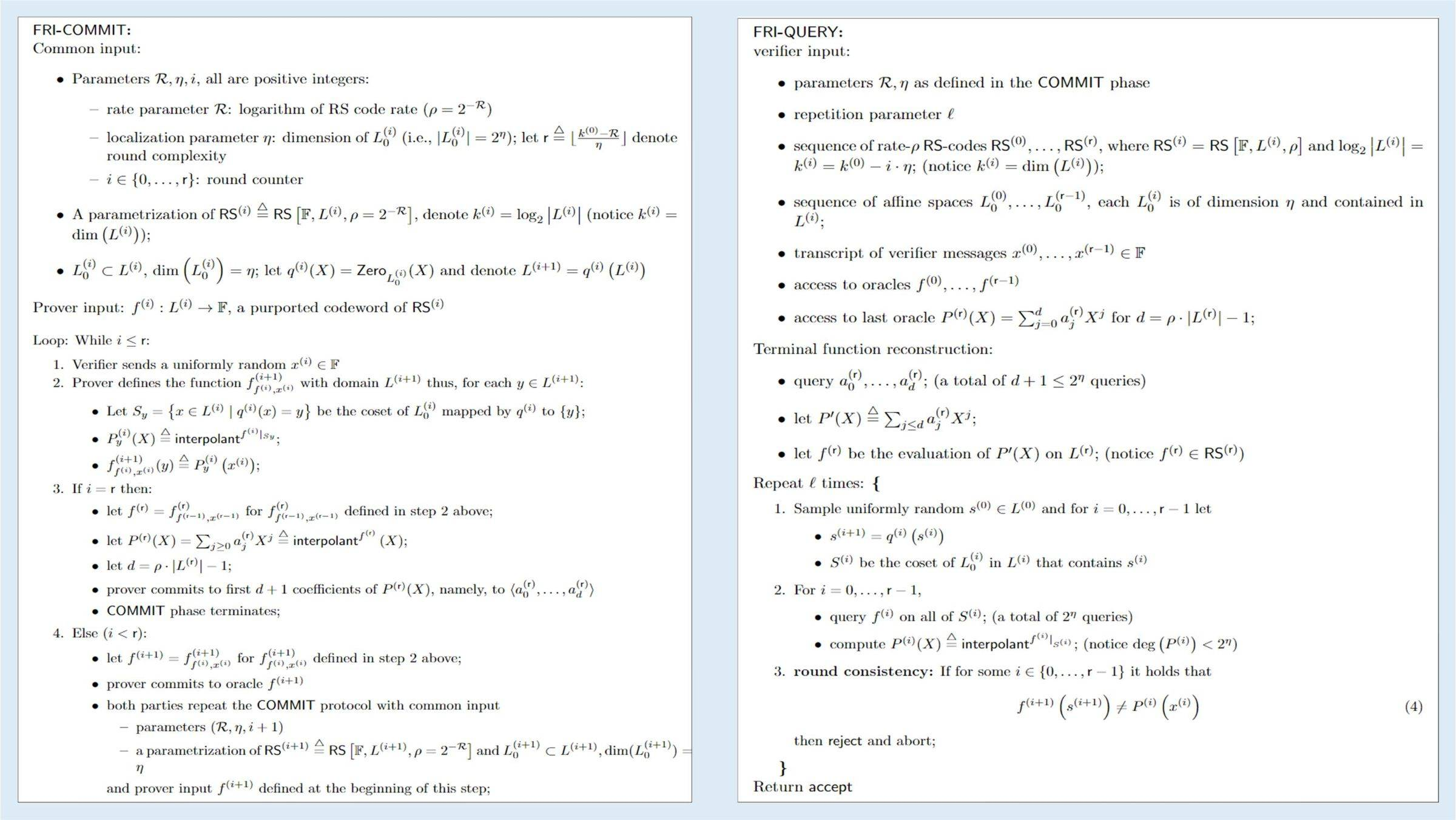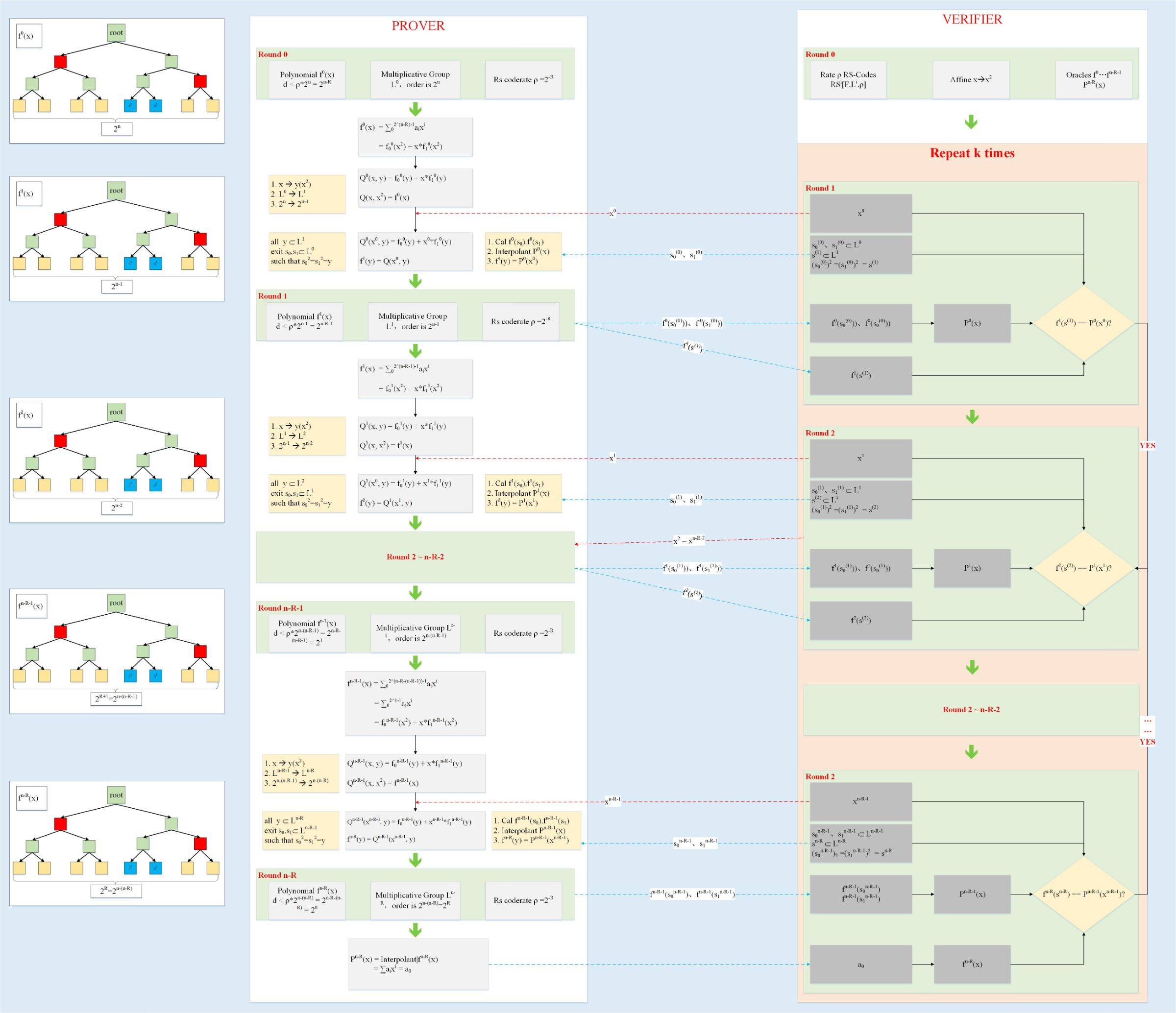## FRI协议

• 在有限域 F 上，存在一个乘法群 L0，群的阶为 2^n；
• 这时，证明者声称码字 f0:L0-->F 是满足 RS[F,L0,ρ] 编码参数的一个码字，即 f0 的大部分点在一个度 d<ρ*2^n 的多项式上 P(x)上，这里 ρ=2^(-R)；

f0(x) = P(x) = P1(x^2) + x * P2(x^2) (1)

f1(y) = Q(x0, y) = P1(y) + x0 * P2(y) (2)

• 群的阶为 2^(n-1);
• 群 L1 的每个元素对应群 L0 的两个元素，即群 L1 的任意 y，群 L0 都有两个 x 和(-x)mod F，满足 x^2 mod F = y && (-x)^2mod F = y；

• 验证者分别从群 L1 和群 L0 选取三个点 y,s0,s1满足 s0!=s1 && s0^2 = s1^2 = y
• 证明者返回 f0(s0),f0(s1),f1(y)三个值
• 验证者根据 f0(s0),f0(s1) 插值出一个关于 x 的 d<2 的多项式 g(x)
• 验证者验证 g(s0) = f1(y)，不相等，则失败FRI 协议分为两个阶段：Commit 阶段和 Query 阶段。从前面简单的场景可以看出，一次简单的循环，需要:

1. 验证者发送随机数 x0
2. 后证明者生成新函数 f1
3. 进行一致性校验

FRI 协议把每一循环前 2 步归类到 Commit 阶段，把第3步归类到了 Query 阶段。即在 Commit 阶段，生成所有的函数 f0~fr，r 为循环的次数，然后在 Query 阶段，统一校验。

Commit：

• Common input
• R RS 编码比率
• i 循环次数索引，取值 {0~r}
• r 循环次数 取值 k0-R/η
• η 空间映射参数 x-->x^(2^η)
• L0 群的阶 2^k0
• RS[F,Li,ρ] 编码参数[ 有限域，作用域，编码比率 ]
• q0(x) = x^(2^η)（实际实现的定义，和图中不一致），L(i+1) = q0(Li)，表示群 Li 到群 L(i+1) 的 2^η --> 1 映射
• Prover input
• fi 第 i 次循环的函数输入
• Li 第 i 次循环的群，阶位 2^(n-i)
• RSi fi 对应的编码参数
• LOOP i <= r
• 1
• xi 验证者发送的随机数
• 2
• Sy 群 L(i+1)的每一个元素对应于群 Li 的元素的集合
• f(i+1)(y) 计算 f(i+1)在群 L(i+1)上的所有取值
• 3 i==r
• 定义 fr 第 2 步的输出
• 插值出 P(x)
• d 是多项式 P(x) 的度
• 保存 d+1 个多项式 P(x) 的系数 a0~ad
• Commit 阶段终止
• 4 i < r
• 定义 f(i+1) 按照第 2 步的计算方式
• 保存 f(i+1)的值，在群 L(i+1)
• 进入下一步循环

Query

• verifier input
• R /η /Li /RSi /xi /fi /P(x) 见Commit
• l query次数
• 重构 fr
• 计算 P`(x)在群 Lr 上的所有取值，并赋值给 fr，注 fr 满足 RSr
• repeat l times
• i = {0~r-1}
• Si 满足 s(i+1) = q0(x)的 x 的集合
• i = {0~r-1}
• 在 Si 上，插值出 Pi(x)
• round consistency check i = {0~r-1}
• f(i+1)(s(i+1)) = Pi(xi)
• 都成功，则验证通过• 针对每一轮的一致性的校验，确保了原始多项式 f0 的确满足 d < ρ*2^n
• 上述协议重复 l 次，可以大大降低作恶者成功的概率

## 总结

1. 算法分为两部分：算术化和 LDT
2. 算术化把问题转换位多项式相等以及多项式的 LDT 问题
3. LDT 阶段使用 FRI 协议，保证线性级的证明复杂度和对数级的验证复杂度
4. 零知识属性保证验证者不能访问轨迹多项式里的点，轨迹多项式里保存着隐私值
5. 同时为了保证零知识属性，需要对轨迹多项式附加数行随机值，由验证者和证明者协商确定
6. 整个过程，不需要第三方的 CRS
7. 整个过程，不依赖任何数学难题

## 附录

• 发表于 2021-02-03 11:37
• 阅读 ( 203 )
• 学分 ( 2 )
• 分类：零知识证明